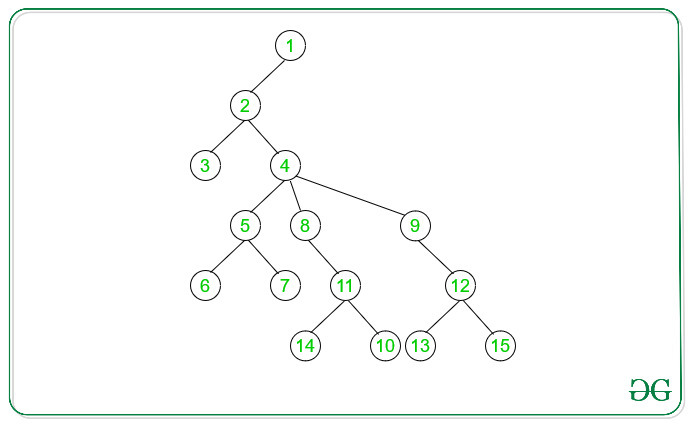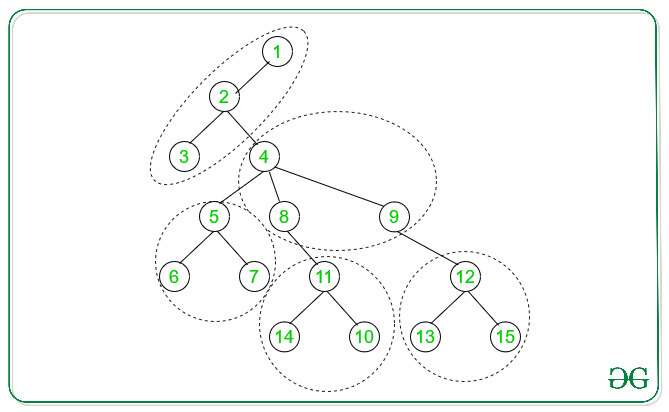# Check if a Tree can be split into K equal connected components

Given Adjacency List representation of a tree and an integer K., the task is to find whether the given tree can be split into K equal Connected Components or not.

Note: Two connected components are said to be equal if they contain equal number of nodes.

Examples:

Input: N = 15, K = 5
Beow is the given tree with Number nodes = 15Output: YES
Explanation:
Below is the 5 number of Connected Components can be made:## Recommended: Please try your approach on {IDE} first, before moving on to the solution.

Approach:
The idea is to use Depth First Search(DFS) traversal on the given tree of N nodes to find whether the given tree can be split into K equal Connected Components or not. Following are the steps:

1. Start DFS Traversal with the root of the tree.
2. For every vertex not visited during DFS traversal, recursively call DFS for that vertex keeping the count of nodes traverse during every DFS recursive call.
3. If the count of nodes is equals to (N/K) then we got our one of the set of Connected Components.
4. If the total number of the set of Connected Components of (N/K) nodes is equal to K. Then the given graph can be split into K equals Connected Components.

Below is the implementation of the above approach:

## C++

 `// C++ program to detect whether ` `// the given Tree can be split ` `// into K equals components ` `#include ` `using` `namespace` `std; ` ` `  `// For checking if the graph ` `// can be split into K equal ` `// Connected Components ` `int` `flag = 0; ` ` `  `// DFS Traversal ` `int` `DFS(vector<``int``> adj[], ``int` `k, ` `        ``int` `i, ``int` `x) ` `{ ` ` `  `    ``// Intialise ans to 1 ` `    ``int` `ans = 1; ` ` `  `    ``// Traverse the adjacency ` `    ``// for vertex i ` `    ``for` `(``auto``& it : adj[i]) { ` `        ``if` `(it != k) { ` `            ``ans += DFS(adj, i, it, x); ` `        ``} ` `    ``} ` ` `  `    ``// If number of nodes is ` `    ``// greater than x, then ` `    ``// the tree cannot be split ` `    ``if` `(ans > x) { ` `        ``flag = 1; ` `        ``return` `0; ` `    ``} ` ` `  `    ``// Check for requirement ` `    ``// of nodes ` `    ``else` `if` `(ans == x) { ` `        ``ans = 0; ` `    ``} ` `    ``return` `ans; ` `} ` ` `  `// A utility function to add ` `// an edge in an undirected ` `// Tree ` `void` `addEdge(vector<``int``> adj[], ` `             ``int` `u, ``int` `v) ` `{ ` `    ``adj[u].push_back(v); ` `    ``adj[v].push_back(u); ` `} ` ` `  `// Driver's Code ` `int` `main() ` `{ ` `    ``int` `N = 15, K = 5; ` ` `  `    ``// Adjacency List ` `    ``vector<``int``> adj[N + 1]; ` ` `  `    ``// Adding edges to List ` `    ``addEdge(adj, 1, 2); ` `    ``addEdge(adj, 2, 3); ` `    ``addEdge(adj, 2, 4); ` `    ``addEdge(adj, 4, 5); ` `    ``addEdge(adj, 5, 6); ` `    ``addEdge(adj, 5, 7); ` `    ``addEdge(adj, 4, 8); ` `    ``addEdge(adj, 4, 9); ` `    ``addEdge(adj, 8, 11); ` `    ``addEdge(adj, 10, 11); ` `    ``addEdge(adj, 11, 14); ` `    ``addEdge(adj, 9, 12); ` `    ``addEdge(adj, 12, 15); ` `    ``addEdge(adj, 12, 13); ` ` `  `    ``// Check if tree can be split ` `    ``// into K Connected Components ` `    ``// of equal number of nodes ` `    ``if` `(N % K == 0) { ` `        ``// DFS call to Check ` `        ``// if tree can be split ` `        ``DFS(adj, -1, 1, N / K); ` `    ``} ` ` `  `    ``// If flag is 0, then the ` `    ``// given can be split to ` `    ``// Connected Components ` `    ``cout << (flag ? ``"NO"` `: ``"YES"``); ` ` `  `    ``return` `0; ` `} `

## Java

 `// Java program to detect whether ` `// the given Tree can be split ` `// into K equals components ` `import` `java.util.*; ` ` `  `class` `GFG ` `{ ` `  `  `// For checking if the graph ` `// can be split into K equal ` `// Connected Components ` `static` `int` `flag = ``0``; ` `  `  `// DFS Traversal ` `static` `int` `DFS(Vector adj[], ``int` `k, ` `        ``int` `i, ``int` `x) ` `{ ` `  `  `    ``// Intialise ans to 1 ` `    ``int` `ans = ``1``; ` `  `  `    ``// Traverse the adjacency ` `    ``// for vertex i ` `    ``for` `(``int` `it : adj[i]) { ` `        ``if` `(it != k) { ` `            ``ans += DFS(adj, i, it, x); ` `        ``} ` `    ``} ` `  `  `    ``// If number of nodes is ` `    ``// greater than x, then ` `    ``// the tree cannot be split ` `    ``if` `(ans > x) { ` `        ``flag = ``1``; ` `        ``return` `0``; ` `    ``} ` `  `  `    ``// Check for requirement ` `    ``// of nodes ` `    ``else` `if` `(ans == x) { ` `        ``ans = ``0``; ` `    ``} ` `    ``return` `ans; ` `} ` `  `  `// A utility function to add ` `// an edge in an undirected ` `// Tree ` `static` `void` `addEdge(Vector adj[], ` `             ``int` `u, ``int` `v) ` `{ ` `    ``adj[u].add(v); ` `    ``adj[v].add(u); ` `} ` `  `  `// Driver's Code ` `public` `static` `void` `main(String[] args) ` `{ ` `    ``int` `N = ``15``, K = ``5``; ` `  `  `    ``// Adjacency List ` `    ``Vector []adj = ``new` `Vector[N + ``1``]; ` `    ``for``(``int` `i= ``0``; i < N + ``1``; i++) ` `        ``adj[i] = ``new` `Vector(); ` `     `  `    ``// Adding edges to List ` `    ``addEdge(adj, ``1``, ``2``); ` `    ``addEdge(adj, ``2``, ``3``); ` `    ``addEdge(adj, ``2``, ``4``); ` `    ``addEdge(adj, ``4``, ``5``); ` `    ``addEdge(adj, ``5``, ``6``); ` `    ``addEdge(adj, ``5``, ``7``); ` `    ``addEdge(adj, ``4``, ``8``); ` `    ``addEdge(adj, ``4``, ``9``); ` `    ``addEdge(adj, ``8``, ``11``); ` `    ``addEdge(adj, ``10``, ``11``); ` `    ``addEdge(adj, ``11``, ``14``); ` `    ``addEdge(adj, ``9``, ``12``); ` `    ``addEdge(adj, ``12``, ``15``); ` `    ``addEdge(adj, ``12``, ``13``); ` `  `  `    ``// Check if tree can be split ` `    ``// into K Connected Components ` `    ``// of equal number of nodes ` `    ``if` `(N % K == ``0``) { ` `        ``// DFS call to Check ` `        ``// if tree can be split ` `        ``DFS(adj, -``1``, ``1``, N / K); ` `    ``} ` `  `  `    ``// If flag is 0, then the ` `    ``// given can be split to ` `    ``// Connected Components ` `    ``System.out.print(flag==``1` `? ``"NO"` `: ``"YES"``);  ` `} ` `} ` ` `  `// This code is contributed by Rajput-Ji `

## C#

 `// C# program to detect whether ` `// the given Tree can be split ` `// into K equals components ` `using` `System; ` `using` `System.Collections.Generic; ` ` `  `class` `GFG ` `{ ` `   `  `// For checking if the graph ` `// can be split into K equal ` `// Connected Components ` `static` `int` `flag = 0; ` `   `  `// DFS Traversal ` `static` `int` `DFS(List<``int``> []adj, ``int` `k, ` `        ``int` `i, ``int` `x) ` `{ ` `   `  `    ``// Intialise ans to 1 ` `    ``int` `ans = 1; ` `   `  `    ``// Traverse the adjacency ` `    ``// for vertex i ` `    ``foreach` `(``int` `it ``in` `adj[i]) { ` `        ``if` `(it != k) { ` `            ``ans += DFS(adj, i, it, x); ` `        ``} ` `    ``} ` `   `  `    ``// If number of nodes is ` `    ``// greater than x, then ` `    ``// the tree cannot be split ` `    ``if` `(ans > x) { ` `        ``flag = 1; ` `        ``return` `0; ` `    ``} ` `   `  `    ``// Check for requirement ` `    ``// of nodes ` `    ``else` `if` `(ans == x) { ` `        ``ans = 0; ` `    ``} ` `    ``return` `ans; ` `} ` `   `  `// A utility function to add ` `// an edge in an undirected ` `// Tree ` `static` `void` `addEdge(List<``int``> []adj, ` `             ``int` `u, ``int` `v) ` `{ ` `    ``adj[u].Add(v); ` `    ``adj[v].Add(u); ` `} ` `   `  `// Driver's Code ` `public` `static` `void` `Main(String[] args) ` `{ ` `    ``int` `N = 15, K = 5; ` `   `  `    ``// Adjacency List ` `    ``List<``int``> []adj = ``new` `List<``int``>[N + 1]; ` `    ``for``(``int` `i= 0; i < N + 1; i++) ` `        ``adj[i] = ``new` `List<``int``>(); ` `      `  `    ``// Adding edges to List ` `    ``addEdge(adj, 1, 2); ` `    ``addEdge(adj, 2, 3); ` `    ``addEdge(adj, 2, 4); ` `    ``addEdge(adj, 4, 5); ` `    ``addEdge(adj, 5, 6); ` `    ``addEdge(adj, 5, 7); ` `    ``addEdge(adj, 4, 8); ` `    ``addEdge(adj, 4, 9); ` `    ``addEdge(adj, 8, 11); ` `    ``addEdge(adj, 10, 11); ` `    ``addEdge(adj, 11, 14); ` `    ``addEdge(adj, 9, 12); ` `    ``addEdge(adj, 12, 15); ` `    ``addEdge(adj, 12, 13); ` `   `  `    ``// Check if tree can be split ` `    ``// into K Connected Components ` `    ``// of equal number of nodes ` `    ``if` `(N % K == 0) { ` `        ``// DFS call to Check ` `        ``// if tree can be split ` `        ``DFS(adj, -1, 1, N / K); ` `    ``} ` `   `  `    ``// If flag is 0, then the ` `    ``// given can be split to ` `    ``// Connected Components ` `    ``Console.Write(flag==1 ? ``"NO"` `: ``"YES"``);  ` `} ` `} ` ` `  `// This code contributed by Rajput-Ji `

Output:

```YES
```

Time Complexity: O(V + E), where V is the number of vertices and E is the number of edges

Attention reader! Don’t stop learning now. Get hold of all the important DSA concepts with the DSA Self Paced Course at a student-friendly price and become industry ready.

My Personal Notes arrow_drop_upCheck out this Author's contributed articles.

If you like GeeksforGeeks and would like to contribute, you can also write an article using contribute.geeksforgeeks.org or mail your article to contribute@geeksforgeeks.org. See your article appearing on the GeeksforGeeks main page and help other Geeks.

Please Improve this article if you find anything incorrect by clicking on the "Improve Article" button below.

Improved By : Rajput-Ji

Article Tags :
Practice Tags :

2

Please write to us at contribute@geeksforgeeks.org to report any issue with the above content.